Prime Factorisation

Chapter 3 Class 6 Playing with Numbers
Concept wise

Prime factorization is a method to write number as multiplication of prime numbers

Let’s take an example

## 24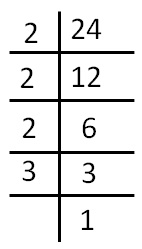24 = 2 × 2 × 2 × 3

## Steps

The steps are

1. Divide the number by 2.
If it is divisible, continue dividing until we cannot further divide or until the number becomes 1
2. If number becomes 1, stop.
3. If the number is not 1, keep on dividing by other prime numbers 3, 5, 7, 11…
4. Stop when number becomes 1

## 12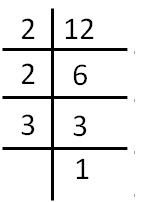12 = 2 × 2 × 3

## 16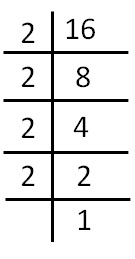16 = 2 × 2 × 2 × 2

## 324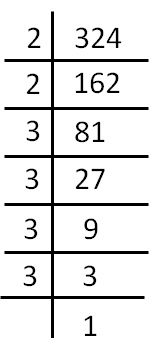324 = 2 × 2 × 3 × 3 × 3 × 3

## 3360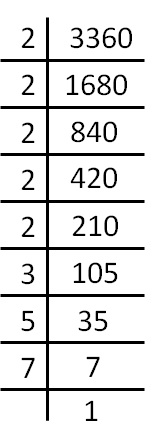3360 = 2 × 2 × 2 × 2 × 2 × 3 × 5 × 7

Note :

In prime factorization, we only include prime numbers.

So, 1 and the number itself is not included in it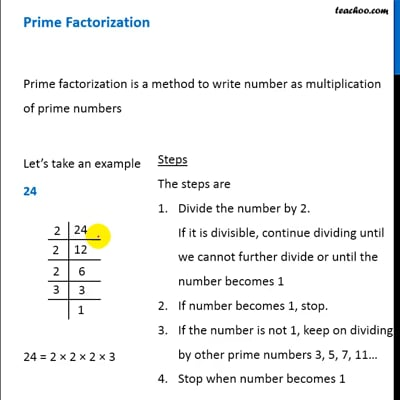This video is only available for Teachoo black users